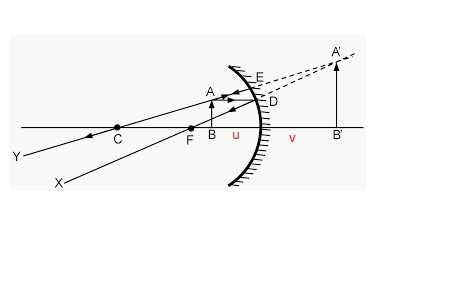# A concave mirror has a radius of curvature of 3.4 cm. (a) Determine the object position for which...

## Question:

A concave mirror has a radius of curvature of 3.4 cm. (a) Determine the object position for which the resulting image is upright and seven times the size of the object. cm? (b) Draw a ray diagram to determine the position of the image. (Use a negative number if the image is behind the mirror.) cm from the mirror?

## Magnification of the mirror:

The magnification is defined as the ratio of the image size formed by the mirror to the original size of the object. It can also be defined as the ratio of the image distance to the object distance. If the magnification is positive, the image will be upright, and if it is negative the image will be inverted.

Given data:

• Radius of curvature of the mirror {eq}\rm (R) = 3.4 \ cm {/eq}
• magnification {eq}\rm (m) = 7 {/eq}

Let the image distance be {eq}v {/eq} and the object distance be {eq}u {/eq}

(a)

Now, the focal length of the mirror would be

{eq}\rm f = \dfrac{R}{2} \\ f = \dfrac{3.4}{2} \\ f = 1.7 \ cm {/eq}

Now, using the magnification of the mirror, we can find the image distance as a function of the object distance

{eq}\rm m = \dfrac{-v}{u} \\ 7 = \dfrac{-v}{u} \\ v = -7u {/eq}

Note, we define m to be positive so that we get an upright image.

Now, using the mirror formula, we can solve for the object distance that results in an upright image that is seven times the size of the object:

{eq}\rm \dfrac{1}{u} + \dfrac{1}{v} = \dfrac{1}{f} \\ \dfrac{1}{u} + \dfrac{1}{-7u} = \dfrac{1}{1.7} \\ u = 1.46 \ cm {/eq}

(b)Since the image is formed behind the lens, therefore the image distance would be negative. And the image distance would be

{eq}\rm v = -10.22 \ cm {/eq}